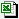# Pricing Models

Darren Webb
Development Team
Montego Data Limited
September 2001-13

The Pricing Models folder under the Listing Map displays a number of Monetary and Equity Derivatives Models
written in Java, more will be added as we complete the library in Java, C++ and Visual Basic For Applications.

Available is Swing Java Jar File: Derivatives Models if you just wish to run the models without page documentation.
Default Security with Java is only to allow trusted code Allowing JavaApplets And JavaSwingJar To Run is needed for this site.

A complete set of models written in Microsoft Excel Visual Basic For Applications can be viewed in the large 438KB file.
Excel VBA ModelsThe ubiquious option formula originates from Black-Scholes 1973
Research of the model over a decade allowed for a generalisation to extend it beyond the vanilla stock option pricing
by the usage of Cost of Carry
In programming terms: price = BlackScholes( pc, S, X, T, V, r, b )
Where pc is Put or Call ; S is Stock or Spot Price ; X is Strike ; T is Time ; V is Volatility ; r is domestic interest rate
and b is Cost of Carry
When
b = r, BlackScholes (1973) Stock Option Model
b = r - q, Merton (1973) Stock Option Model - continuous dividend yield q
b = 0, Black (1976) Futures Option Model
b = r - rf, Garman-Kohlhagen (1983) Currency Option Model

Note these are Closed-Form Solutions and the general rule is that they price European Options.
That is, they are assumed to follow the Brownian stochastic process
which is an assumption that the underlying asset is lognormally (geometrically) distributed.

The mathematical formula is as follows:
cBlack-Scholes = Se(b-r)TN(d1) - Xe-rTN(d2)

pBlack-Scholes = Xe-rTN(-d2) - Se(b-r)TN(-d1)

Where e is exp(), σ is volatility
and d1 is ( ln(S/X) + (b+σ2/2)T ) / σsqrt(T)
and d2 is ( ln(S/X) + (b-σ2/2)T ) / σsqrt(T) = d1 - σsqrt(T)

As a computer algorithm in the C programming language:
double BlackScholes( char CallPutFlag, double S, double X, double T, double r, double b, double v )
{
double d1, d2, bs = 0.0;

d1 = (log(S / X) + (b + pow(v,2) / 2.0) * T) / (v * sqrt(T));
d2 = d1 - v * sqrt(T);

if ( CallPutFlag == 'C' )
bs = S * exp((b - r) * T) * CND(d1) - X * exp(-r * T) * CND(d2);
else if ( CallPutFlag == 'P' )
bs = X * exp(-r * T) * CND(-d2) - S * exp((b - r) * T) * CND(-d1);

return bs;
}
It should be recognised when using Black-76 to price Interest Rate Options:
1. a) The underlying asset is lognormally distributed
2. b) With a cap the underlying Forward/Future rates are assumed to be lognormal
3. c) With a swaption (option on a swap) the underlying swap rate is assumed to be lognormal
As such a simultaneous valuation of both a cap and a swaption with the Black-76 model is theoretically inconsistant.
Both the forward rate of the cap and the swap rate cannot be lognormal simultaneously.
This also applies to bond prices and swap rates: they cannot be lognormal at the same time.
If the bond price is assumed to be lognormal then the continuously compounded swap rate must be normally distributed.
The practical workaround is to adjust the volatility to kludge the option price based on trader experience.

Finally this leads to the Cumulative Normal Distribution [CND() - not shown here] function
This can be found in most text books. It is possible to use as little as four digit accuracy but it is much better to use six digit accuracy. Note 1 / 64 = 0.015625.
Professor Mark Garman in the 1980's recommended a CND() function to seven digits for Monetary Derivatives.

```Public Function GDelta(CallPutFlag As String, S As Double, X As Double, T As Double, r As Double, b As Double, v As Double) As Double

Dim d1 As Double

d1 = (Log(S / X) + (b + v ^ 2 / 2) * T) / (v * Sqr(T))

If CallPutFlag = "c" Then
GDelta = Exp((b - r) * T) * CND(d1)
ElseIf CallPutFlag = "p" Then
GDelta = Exp((b - r) * T) * (CND(d1) - 1)
End If
End Function
```

Δ Delta (Asset)   Γ Gamma (delta)   θ Theta (time)    σ Vega (sigma)    Ρ Rho (yield)